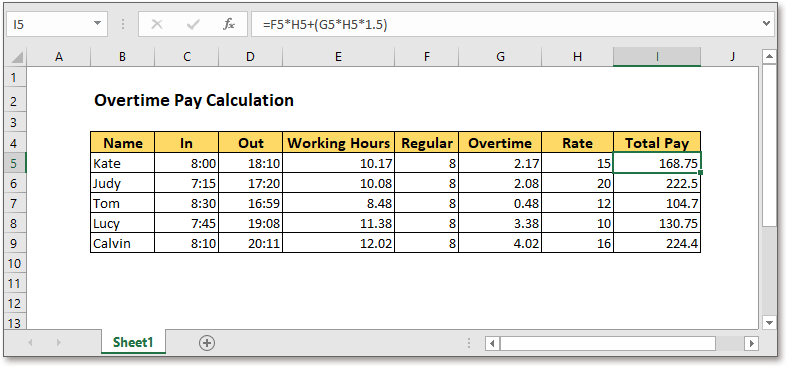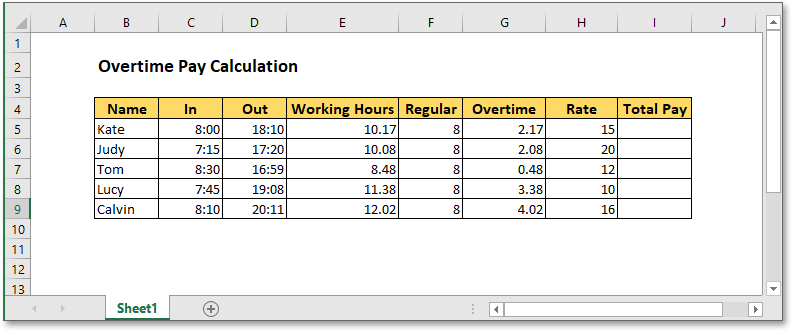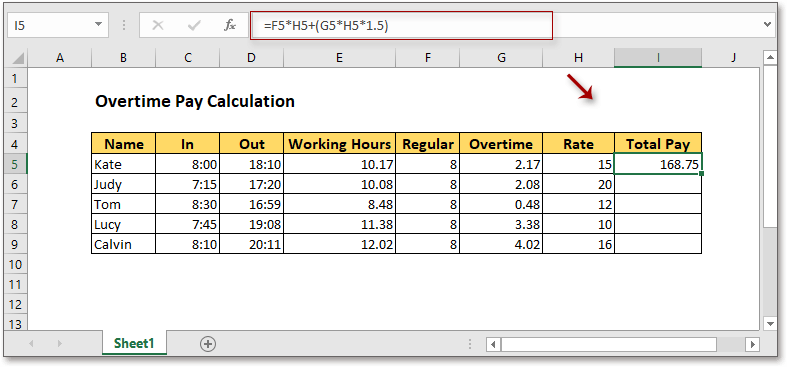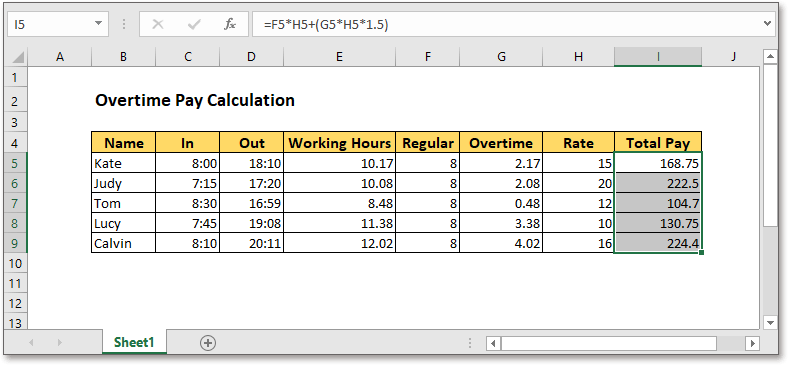## Excel Formula: Calculate overtime payGenerally, overtime pay is different from the regular working pay, for instance, 10 dollars per hour for regular working hours, but 15 dollars per hour for working overtime. Here in this tutorial, it shows a timesheet to list the working hours, and provides a formula to calculate the overtime pay.Generic formula:

 (reg_hrs*rate)+(ot_hrs*rate*1.5)

Arguments

 Reg_hrs: the number of regular working hours. Ot_hrs: the number of overtime working hours. Rate: regular hourly pay. 1,5: 1.5 times the normal rate. Here taking into account overtime paid at 1.5 times the normal rate. You can change it to the actual value.

Return value

It returns the total pay including regular working pay and overtime working pay.

How this formula workSupposing the timesheet is shown as above, please use the formula as this:

 =F5*H5+(G5*H5*1.5)

Press Enter key, the total pay has been calculated.Then drag the fill handle over the cells you want to calculate the pay.Note

Calculate the working hours: =(outtime-intime)*24, for instant, =(D5-C5)*24

Calculate the overtime hours: =workinghours-regular, for instant, =E5-F5